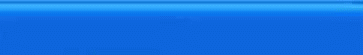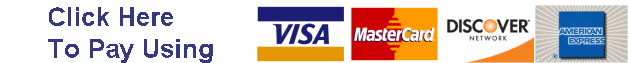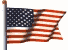HomeEmail: superlumination@cox.netSearch

How much light does the LED bulb produce?

The simple answer is: Generally not as much as its incandescent counterpart and never enough.

While LED bulbs are generally more intense than incandescent counterparts, the incandescent bulbs are perceived as being “brighter” in stock lens housings because the light is emitted in all directions and is refocused and reflected by the reflectors in the back of the lens housings.

If you really want to understand the gory details, they are explained below as comprehensively and yet as simple as I have been able to compile the information:

______________________________________________________________________

First some definitions (right out of Websters):

A.  Main Entry: hyper-
Function: prefix
Etymology: Middle English iper-, from Latin hyper-, from Greek, from hyper -- more at
OVER
1 : above : beyond : SUPER- < hypermarket>
2 a : excessively < hypersensitive> b : excessive < hyperemia>
3 : that is or exists in a space of more than three dimensions < hyperspace>

The term “Hyper” is a basically meaningless prefix.  It can only be used to compare one thing to another, so to claim that a bulb is “hyper”, you need to ask hyper as compared to what?

B. Main Entry: 1su·per
Pronunciation:
'sü-p&r
Etymology: super-
Date: 1837
1 a -- used as a generalized term of approval <a super cook> b : of high grade or quality
2 : very large or powerful <a super atomic bomb>
3 : exhibiting the characteristics of its type to an extreme or excessive degree < super secrecy>

The term “Super” is a basically meaningless adjective.  It can only be used to compare one thing to another, so to claim that something is “super”, is pretty much just an opinion.

C.  Main Entry: bright·ness
Function: noun
Date: before 12th century
1 a : the quality or state of being bright; also : an instance of such a quality or state.
2 : the attribute of light-source colors by which emitted light is ordered continuously from light to dark in correlation with its intensity

The term “brightness” has no units of measure, and therefore cannot be used for scientific comparison.  “Hyper” and “Super” are even less scientific.

These are all subjective term that has no unit of measure and is not scientifically specified in numerical terms.  Regardless, most people describe and compare light and light bulbs in terms of brightness.  In most peoples mind, brightness refers to how well they can visually perceive the light from wherever they happen to be standing.  A person’s perception of brightness is a function of many different things:

• Color

• Viewing angle

• Distance from the light source

• Area of illumination

• Lens & reflector housings

D. Watts

Watts is a measure of power or energy consumed, not “brightness”.    In the world of incandescent lighting, you can often use watts as a comparison of how “bright” one light bulb is compared to another light bulb of the same style because the one that uses more power will generally be perceived as being “brighter”.    Using watts to compare one style of bulb (such as incandescent) to another style (like led) is meaningless, since LEDS are about 5 times more efficient at converting energy to light as compared to incandescent bulbs.

LED light output is often rated in candela (cd), or thousandths of a cd (mcd or millicandelas). For example, our 3157 12 led super white bulbs are rated at 79000 mcd.

A basic General Electric 60W light bulb, for example, is rated at ~850 lumens.

If you could compare the LEDS to something familiar like a regular light bulb, you'd have a grasp of how much light the LED produces. ______________________________________________________________________

So how do you measure and compare how much light a light source emits?  How can you compare LEDS to incandescent bulbs?

You need some additional information on the candela (candlepower) and the lumen, and something called a solid angle, which is measured in steradians

"One steradian (sr) is the solid angle that, having its vertex in the center of a sphere, cuts off an area on the surface of the sphere equal to that of a square with sides of length equal to the radius of the sphere."

The solid angle is thus ratio of the spherical area to the square of the radius. The spherical area is a projection of the object of interest onto a unit sphere, and the solid angle is the surface area of that projection. If we divide the surface area of a sphere by the square of its radius, we find that there are 4 p steradians of solid angle in a sphere. One hemisphere has 2p  steradians.

The symbol for solid angle is either w , the lowercase Greek letter omega, or W , the uppercase omega.

A complete sphere measures 4 p  steradians ( W).

p is a constant that = 22/7=~3.14

Candela (cd) Iv

The candela is one of the seven base unts of the SI system.  It is defined as follows:

The candela is the luminous intensity, in a given direction, of a source that emits monochromatic radiation of frequency 540 × 1012 hertz and that has a radiant intensity in that direction of 1/683 watt per steradian.

The candela is abbreviated as cd and its symbol is Iv.

The candela is a measure of the intensity of the light source and is sometimes commonly called brightness.

L umen (lm) Fv.

The lumen is an SI derived unit for luminous flux.   The abbreviation is lm and the symbol is Fv. The lumen is derived from the candela and is the luminous flux emitted into unit solid angle (1 sr) by an isotropic point source having a luminous intensity of 1 candela.   The lumen is the product of luminous intensity and solid angle, cd-sr.  It is analogous to the unit of radiant flux (watt), differing only in the eye response weighting.  If a light source is isotropic, the relationship between lumens and candelas is 1 cd = 4p lm. In other words, an isotropic source having a luminous intensity of 1 candela emits 4p lumens into space, which just happens to be 4p steradians. We can also state that 1 cd = 1 lm/sr, analogous to the equivalent radiometric definition.

The lumen is a measure of total light output and is also often confused with intensity and is also sometimes commonly called brightness.

Luminance is another SI derived quantity which denotes luminous flux density . It has a special name, lux, and is lumens per square metre, or lm/m2. The symbol is Ev.

Luminance is probably the best overall measure of what most people commonly refer to as “brightness”.  It can only be determined from the bulb in combination with a particular object to be illuminated such as a tail light lens.  The same bulb may provide more illumination in one taillight assembly than the same bulb will provide in a different taillight assembly.  Because of this, we cannot produce illumination data for every bulb in every vehicle and every type of lens.

1 metre-candle = 1 lux
1 phot = 1 lm/cm2 = 104 lux
1 foot-candle = 1 lumen/ft2 = 10.76 lux
1 milliphot = 10 lux

A candela is the intensity of a light source that gives off one lumen per steradian, that is, 4 p lumens.

or (4 x 3.14 = 12.57 Lumens).

So:

1 candela (cd) = 1 candlepower (cp)= 4 p   lumens = (4 x 3.14) = 12.57 lumens

M = Light Output (millicandella)

Iv = Intensity or candlepower (candela)

F v = Total Light Output (lumens)

M=1000 x Iv

F v = Iv x 12.57      (For a spherical light source only)

Using that formula, you would  think that a 2300 mcd LED gives off:

2300 millicandellas = 2.3 candelas x 12.57 lumens/candelas= 28.66 lumens

28.66/865 = 3.3% as much light as a 60 watt light bulb.

That's about 1/30 of the 60W light bulb, or in the same ballpark as a 2-1/2 watt bike headlight, using less than 1/10 of a watt of electricity. That would be an impressive LED, all right, but alas, those things that sound too good to be true....

All lights do not emit light in a spherical pattern.

A regular light bulb emits light in an almost complete spherical pattern.

LEDS emit light only in certain directions called beam angles.

If the LED looked like 2.3 cd (candlepower) no matter where you look at it from--that is, from anywhere on an imaginary sphere around it, then you could call it a 2.3 spherical candlepower (SCP) light source. Then it would really be 2.3 spherical candlepower and  would indeed be giving off 29 lumens.

It would still be 2.3 SCP.  If it were brighter from some directions than others, as long as the average (mean) were 2.3 cd over an entire surrounding sphere. Some light bulbs are rated this way, in mean spherical candlepower (MSCP).

For incandescent bulbs:

F v = MSCP x 12.57

The typical LED throws a narrower beam in one direction.  If it is rated 2.3 cd, that means if you look at it from anywhere within that beam angle, you are looking at a 2.3 cd light source. From anywhere else, you are looking at a dim plastic blob with wires. It has 2.3 beam candlepower, but not 2.3 spherical candlepower, and the total light being produced is much less than 29 lumens.

Calculating Lumens from Candela

If you want to know the total output in lumens of an LED, you need two things: the candela rating in the beam (2.3 cd in the example) and also the solid angle of the beam (beam angle).

The solid angle indicates what portion of the surface area of an enclosing unit sphere would be illuminated by the led light beam. Find the illuminated area, and that number is also the solid angle in steradians.

Multiply the candela rating by the steradians to get the lumen output.

Total light output (lumens) = Intensity (candela) x Solid Angle (steradians)

F v = Total Light Output (lumens)

Iv = Intensity (candela)

F v = Iv x W

If your light bulb lights up the whole unit sphere it has a 360 degree beam angle. The surface area is 4 pi square units and the solid angle is 4 pi steradians.  In this case to convert from spherical candlepower to lumens, you can just multiply by 4 pi or (12.57).

Most LED beam angles are not rated as a solid angle in steradians, but rather a beam angle in degrees.

The beam shape is pretty much a simple circular cone.

To convert beam angle to steradians use the following formula:

B = Beam angle (degrees)

W = (1- Cosine(1/2 x B)) x 2Pi

W = (1- Cosine(1/2 x B)) x 6.28

Now you can multiply the steradians by the candela rating to get an approximation of the LED's output in lumens.

The answer is approximate both because the LED's beam is not exactly the same brightness everywhere within the beam, and also because the beam doesn't really have sharp edges and total darkness around it. But at least you now have something to go on when comparing different LEDS to each other, or comparing an LED to something else you know.

Total light output (lumens) = Intensity (candela) x Solid Angle (steradians)

F v = Total Light Output (lumens)

Iv = Intensity (candela)

B = Beam angle (degrees)

The final formula is :

F v = Iv x [(1- Cosine(1/2 x B)) x 6.28]

Example 1.

1. Calculate the light output of the 3157 12led red led bulb that has an intensity of 77000 mcd and a 30 degree beam angle.

2. Calculate the light output of the 3157 15led red led bulb that has an intensity of 96,000 mcd and a 45 degree beam angle.

3. Calculate the light output of the 3157 19led red led bulb that has an intensity of 122,000 mcd and a 22 degree beam angle.

4. Calculate the light output of the 3157  20led red led bulb that has an intensity of 129,000 mcd and a 45 degree beam angle.

5. Calculate the light output of the 3157 25 watt clear incandescent bulb that has an intensity of 32 mscp.

6. Which one produces more light?

7. Which one is the most intense?

8. Which bulb is the brightest?

9. Which bulb consumes the most power?

Solution.

A. First find the total light output of the 3157 red 12 led bulb:

B=30 degrees

Iv = 77 cd

F v = I x [(1- Cosine(1/2 x B)) x 6.28]

F v = 77 x [(1-Cosine(1/2 x 30)) x 6.28]

F v = 77 x [(1-.966) x 6.28] = 16 lumens

B.  Now find the total light output of the 3157 red 15 led bulb:

B=45 degrees

Iv = 96 cd

F v = I x [(1- Cosine(1/2 x B)) x 6.28]

F v = 96 x [(1-Cosine(1/2 x 45)) x 6.28]

F v = 96 x [(1-.924) x 6.28] = 45 lumens

C.  Now find the total light output of the 3157 red 19 led bulb:

B=35 degrees

Iv = 122 cd

F v = I x [(1- Cosine(1/2 x B)) x 6.28]

F v = 122 x [(1-Cosine(1/2 x 35)) x 6.28]

F v = 122 x [(1-.954) x 6.28] = 35 lumens

D.  Now find the total light output of the 3157 red 20 led bulb:

B=45 degrees

Iv = 129 cd

F v = I x [(1- Cosine(1/2 x B)) x 6.28]

F v = 129 x [(1-Cosine(1/2 x 45)) x 6.28]

F v = 129 x [(1-.923) x 6.28] = 62 lumens

E.  Now find the total light output of the 25 watt 3157 clear incandescent bulb:

B=360 degrees

MSCP = 32 cd

F v = MSCP x 12.46

F v = 32cd x 12.57 lumens/cd = 402 lumens

F.  The 25 watt incandescent bulb produces the most total light output 402 lumens.

G.  The most intense bulb is the 20 led 3157 at 129,000 mcd.

H.  This cannot be answered since brightness has no units for scientific measurement and comparison.

I.

1. 3157 red 12led:(85ma)

Watts = Volts x Amps

Watts = 12 Volts  x .085 Amps = 1 Watt

2. 3157 red 15led:(106ma)

Watts = Volts x Amps

Watts = 12 Volts  x .106 Amps = 1.3 Watt

3. 3157 red 19led:(140ma)

Watts = Volts x Amps

Watts = 12 Volts  x .140 Amps = 1.7 Watt

4. 3157 red 20led:(148ma)

Watts = Volts x Amps

Watts = 12 Volts  x .148 Amps = 1.8 Watt

5. 3157 incandescent 25 watt :(2100ma)

Watts = Volts x Amps

Watts = 12 Volts  x 2.1 Amps = 25 Watt

Caution.

Because intensity isn't exactly the same everywhere inside the beam, and the beam edges aren't really sharp, the calculations shown are approximate.

The point of the calculations is to make informed judgments from the published specs before placing an order.Standard Rates (US Only)

*Via US Postal First Class Mail

* Orders weighing more than 1 lb will ship via parcel post unless special shipping options are added (below).

Shipping & Handling

 Total Order Cost \$.01-\$9.99 \$2.00 \$10.00-\$49.99 \$3.00 \$50.00-\$99.99 \$5.00 \$100-\$199.99 \$10.00 \$200+ \$15.00

*Note: If you do not add any special shipping options to your order, we will automatically ship via US postal first class mail.

You must choose a special shipping option (see below) in order to get a tracking number.

All International orders must choose an international shipping option.UPIC Shipping Insurance

Note: Our packages are not automatically insured against loss during shipping.  Insurance is the responsibility of the purchaser!
\$2.00 for each \$100 unit of insurance

Shipping Insurance

\$2.00 per \$100